# Difference-in-Differences with a Continuous Treatment

Brantly Callaway

University of Georgia

Andrew Goodman-Bacon

Federal Reserve Bank of Minneapolis

Pedro Sant’Anna

Emory University

## What’s Been Happening in the DID Literature?

$$\newcommand{\E}{\mathbb{E}} \newcommand{\E}{\mathbb{E}} \newcommand{\var}{\mathrm{var}} \newcommand{\cov}{\mathrm{cov}} \newcommand{\Var}{\mathrm{var}} \newcommand{\Cov}{\mathrm{cov}} \newcommand{\Corr}{\mathrm{corr}} \newcommand{\corr}{\mathrm{corr}} \newcommand{\L}{\mathrm{L}} \renewcommand{\P}{\mathrm{P}} \newcommand{\independent}{{\perp\!\!\!\perp}} \newcommand{\indicator}{ \mathbf{1}\{#1\} }$$ There have been a number of recent advances in the differences-in-differences literature. Two broad contributions:

• Contribution 1: Diagnose issues with commonly used two-way fixed effects (TWFE) regressions commonly used to implement DID identification strategies $Y_{it} = \theta_t + \eta_i + \beta^{twfe} D_{it} + e_{it}$
• Roughly: TWFE regression can deliver poor estimates of causal effect parameters in the presence of treatment effect heterogeneity
• Contribution 2: Propose alternative estimation strategies that “work” when the identification stratgey works (and are robust to treatment effect heterogeneity)

## This Paper

These papers have (largely) focused on the case with a binary, staggered treatment

Current paper: Move from a setting with a binary treatment case to one with a continuous treatment (“dose”)

Some of the arguments involve extending ideas from the binary, staggered treatment case to a setting with continuous treatment

• But we will also face new conceptual issues in this case that do not show up in a setting with a binary treatment

Example:

• Effect of $$\underbrace{\textrm{length of school closures}}_{\textrm{continuous treatment}}$$ (during Covid) on $$\underbrace{\textrm{students' test scores}}_{\textrm{outcome}}$$

## This paper

For today, mostly emphasize a continuous treatment, but our results also apply to other settings (with trivial modifications):

• multi-valued treatments (e.g., effect of state-level minimum wage policies on employment)

• binary treatment with differential “exposure” to the treatment (application in the paper: a binary Medicare policy where different hospitals had more exposure to the treatment)

But results do not apply to “fuzzy” DID setups

• Fuzzy DID refers to a setting where a researcher is ultimately interested in understanding the effect of a binary treatment but observes aggregate data (e.g., interested in learning about union wage-premium (at individual level) using state-level data and exploiting variation in the “amount” of unionization across different locations)

## Today’s Talk

1. Identification: What’s the same as in the binary treatment case?

2. Identification: What’s different from the binary treatment case?

# Identification: What’s the same as in the binary treatment case?

## Continuous Treatment Notation

Potential outcomes notation

• Two time periods: $$t^*$$ and $$t^*-1$$

• No one treated until period $$t^*$$
• Some units remain untreated in period $$t^*$$
• Potential outcomes: $$Y_{it^*}(d)$$

• Observed outcomes: $$Y_{it^*}$$ and $$Y_{it^*-1}$$

$Y_{it^*}=Y_{it^*}(D_i) \quad \textrm{and} \quad Y_{it^*-1}=Y_{it^*-1}(0)$

## Parameters of Interest (ATT-type)

Level Effects (Average Treatment Effect on the Treated)

$ATT(d|d) := \E[Y_{t^*}(d) - Y_{t^*}(0) | D=d]$

• Interpretation: The average effect of dose $$d$$ relative to not being treated local to the group that actually experienced dose $$d$$

• This is the natural analogue of $$ATT$$ in the binary treatment case

## Parameters of Interest (ATT-type)

Slope Effects (Average Causal Response on the Treated)

$ACRT(d|d) := \frac{\partial ATT(l|d)}{\partial l} \Big|_{l=d}$

• Interpretation: $$ACRT(d|d)$$ is the causal effect of a marginal increase in dose local to units that actually experienced dose $$d$$

We can view $$ACRT(d|d)$$ as the “building block” here. An aggregated version of it (into a single number) is \begin{align*} ACRT^O := \E[ACRT(D|D)|D>0] \end{align*}

• $$ACRT^O$$ averages $$ACRT(d|d)$$ over the population distribution of the dose

• Like $$ATT^O$$ for staggered treatment adoption, $$ACRT^O$$ is the natural target parameter for the TWFE regression in this case

## Identification

“Standard” Parallel Trends Assumption

For all $$d$$,

$\E[\Delta Y_{t^*}(0) | D=d] = \E[\Delta Y_{t^*}(0) | D=0]$

## Identification

“Standard” Parallel Trends Assumption

For all $$d$$,

$\E[\Delta Y_{t^*}(0) | D=d] = \E[\Delta Y_{t^*}(0) | D=0]$

Then,

\begin{aligned} ATT(d|d) &= \E[Y_{t^*}(d) - Y_{t^*}(0) | D=d] \hspace{150pt} \end{aligned}

## Identification

“Standard” Parallel Trends Assumption

For all $$d$$,

$\E[\Delta Y_{t^*}(0) | D=d] = \E[\Delta Y_{t^*}(0) | D=0]$

Then,

\begin{aligned} ATT(d|d) &= \E[Y_{t^*}(d) - Y_{t^*}(0) | D=d] \hspace{150pt}\\ &= \E[Y_{t^*}(d) - Y_{t^*-1}(0) | D=d] - \E[Y_{t^*}(0) - Y_{t^*-1}(0) | D=d] \end{aligned}

## Identification

“Standard” Parallel Trends Assumption

For all $$d$$,

$\E[\Delta Y_{t^*}(0) | D=d] = \E[\Delta Y_{t^*}(0) | D=0]$

Then,

\begin{aligned} ATT(d|d) &= \E[Y_{t^*}(d) - Y_{t^*}(0) | D=d] \hspace{150pt}\\ &= \E[Y_{t^*}(d) - Y_{t^*-1}(0) | D=d] - \E[Y_{t^*}(0) - Y_{t^*-1}(0) | D=d]\\ &= \E[Y_{t^*}(d) - Y_{t^*-1}(0) | D=d] - \E[\Delta Y_{t^*}(0) | D=0] \end{aligned}

## Identification

“Standard” Parallel Trends Assumption

For all $$d$$,

$\E[\Delta Y_{t^*}(0) | D=d] = \E[\Delta Y_{t^*}(0) | D=0]$

Then,

\begin{aligned} ATT(d|d) &= \E[Y_{t^*}(d) - Y_{t^*}(0) | D=d] \hspace{150pt}\\ &= \E[Y_{t^*}(d) - Y_{t^*-1}(0) | D=d] - \E[Y_{t^*}(0) - Y_{t^*-1}(0) | D=d]\\ &= \E[Y_{t^*}(d) - Y_{t^*-1}(0) | D=d] - \E[\Delta Y_{t^*}(0) | D=0]\\ &= \E[\Delta Y_{t^*} | D=d] - \E[\Delta Y_{t^*} | D=0] \end{aligned}

This is exactly what you would expect

# Identification: What’s different from the binary treatment case?

## Are we done?

Unfortunately, no

Most empirical work with a continuous treatment wants to think about how causal responses vary across dose

• Plot treatment effects as a function of dose and ask: does more dose tends to increase/decrease/not affect outcomes?
• Average causal response parameters inherently involve comparisons across slightly different doses

There are new issues related to comparing $$ATT(d|d)$$ at different doses and interpreting these differences as causal effects

• At a high-level, these issues arise from a tension between empirical researchers wanting to use a quasi-experimental research design (which delivers “local” treatment effect parameters) but (often) wanting to compare these “local” parameters to each other

• Unlike the staggered, binary treatment case: No easy fixes here!

## Interpretation Issues

Consider comparing $$ATT(d|d)$$ for two different doses

\begin{aligned} & ATT(d_h|d_h) - ATT(d_l|d_l) \hspace{350pt} \end{aligned}

## Interpretation Issues

Consider comparing $$ATT(d|d)$$ for two different doses

\begin{aligned} & ATT(d_h|d_h) - ATT(d_l|d_l) \hspace{350pt}\\ & \hspace{25pt} = \E[Y_{t^*}(d_h)-Y_{t^*}(d_l) | D=d_h] + \E[Y_{t^*}(d_l) - Y_{t^*}(0) | D=d_h] - \E[Y_{t^*}(d_l) - Y_{t^*}(0) | D=d_l] \end{aligned}

## Interpretation Issues

Consider comparing $$ATT(d|d)$$ for two different doses

\begin{aligned} & ATT(d_h|d_h) - ATT(d_l|d_l) \hspace{350pt}\\ & \hspace{25pt} = \E[Y_{t^*}(d_h)-Y_{t^*}(d_l) | D=d_h] + \E[Y_{t^*}(d_l) - Y_{t^*}(0) | D=d_h] - \E[Y_{t^*}(d_l) - Y_{t^*}(0) | D=d_l]\\ & \hspace{25pt} = \underbrace{\E[Y_{t^*}(d_h) - Y_{t^*}(d_l) | D=d_h]}_{\textrm{Causal Response}} + \underbrace{ATT(d_l|d_h) - ATT(d_l|d_l)}_{\textrm{Selection Bias}} \end{aligned}

“Standard” Parallel Trends is not strong enough to rule out the selection bias terms here

• Implication: If you want to interpret differences in treatment effects across different doses, then you will need stronger assumptions than standard parallel trends

• This problem spills over into identifying $$ACRT(d|d)$$

## Interpretation Issues

Intuition:

• Difference-in-differences identification strategies result in $$ATT(d|d)$$ parameters. These are local parameters and difficult to compare to each

• This explanation is similar to thinking about LATEs with two different instruments

• Thus, comparing $$ATT(d|d)$$ across different values is tricky and not for free

What can you do?

• One idea, just recover $$ATT(d|d)$$ and interpret it cautiously (interpret it by itself not relative to different values of $$d$$)

• If you want to compare them to each other, it will come with the cost of additional (structural) assumptions

## Introduce Stronger Assumptions

“Strong” Parallel Trends Assumption

For all doses d and l,

$\mathbb{E}[Y_{t^*}(d) - Y_{t^*-1}(0) | D=l] = \mathbb{E}[Y_{t^*}(d) - Y_{t^*-1}(0) | D=d]$

• This is notably different from “Standard” Parallel Trends

• It involves potential outcomes for all values of the dose (not just untreated potential outcomes)

• All dose groups would have experienced the same path of outcomes had they been assigned the same dose

## Introduce Stronger Assumptions

Strong parallel trends implies a version of treatment effect homogeneity. Notice:

\begin{aligned} ATT(d|d) &= \E[Y_{t^*}(d) - Y_{t^*}(0) | D=d] \hspace{200pt} \ \end{aligned}

## Introduce Stronger Assumptions

Strong parallel trends implies a version of treatment effect homogeneity. Notice:

\begin{aligned} ATT(d|d) &= \E[Y_{t^*}(d) - Y_{t^*}(0) | D=d] \hspace{200pt} \\\ &= \E[Y_{t^*}(d) - Y_{t^*-1}(0) | D=d] - \E[Y_{t^*}(0) - Y_{t^*-1}(0) | D=d] \ \end{aligned}

## Introduce Stronger Assumptions

Strong parallel trends implies a version of treatment effect homogeneity. Notice:

\begin{aligned} ATT(d|d) &= \E[Y_{t^*}(d) - Y_{t^*}(0) | D=d] \hspace{200pt} \\\ &= \E[Y_{t^*}(d) - Y_{t^*-1}(0) | D=d] - \E[Y_{t^*}(0) - Y_{t^*-1}(0) | D=d] \\\ &= \E[Y_{t^*}(d) - Y_{t^*-1}(0) | D=l] - \E[Y_{t^*}(0) - Y_{t^*-1}(0) | D=l] \ \end{aligned}

## Introduce Stronger Assumptions

Strong parallel trends implies a version of treatment effect homogeneity. Notice:

\begin{aligned} ATT(d|d) &= \E[Y_{t^*}(d) - Y_{t^*}(0) | D=d] \hspace{200pt} \\\ &= \E[Y_{t^*}(d) - Y_{t^*-1}(0) | D=d] - \E[Y_{t^*}(0) - Y_{t^*-1}(0) | D=d] \\\ &= \E[Y_{t^*}(d) - Y_{t^*-1}(0) | D=l] - \E[Y_{t^*}(0) - Y_{t^*-1}(0) | D=l] \\\ &= \E[Y_{t^*}(d) - Y_{t^*}(0) | D=l] = ATT(d|l) \end{aligned}

Since this holds for all $$d$$ and $$l$$, it also implies that $$ATT(d|d) = ATE(d) = \E[Y_{t^*}(d) - Y_{t^*}(0)]$$. Thus, under strong parallel trends, we have that

$ATE(d) = \E[\Delta Y_{t^*}|D=d] - \E[\Delta Y_{t^*}|D=0]$

RHS is exactly the same expression as for $$ATT(d|d)$$ under “standard” parallel trends, but here

• assumptions are different

• parameter interpretation is different

## Comparisons across dose

ATE-type parameters do not suffer from the same issues as ATT-type parameters when making comparisons across dose

\begin{aligned} ATE(d_h) - ATE(d_l) &= \E[Y_{t^*}(d_h) - Y_{t^*}(0)] - \E[Y_{t^*}(d_l) - Y_{t^*}(0)] \end{aligned}

## Comparisons across dose

ATE-type parameters do not suffer from the same issues as ATT-type parameters when making comparisons across dose

\begin{aligned} ATE(d_h) - ATE(d_l) &= \E[Y_{t^*}(d_h) - Y_{t^*}(0)] - \E[Y_{t^*}(d_l) - Y_{t^*}(0)]\\ &= \underbrace{\E[Y_{t^*}(d_h) - Y_{t^*}(d_l)]}_{\textrm{Causal Response}} \end{aligned}

Thus, recovering $$ATE(d)$$ side-steps the issues about comparing treatment effects across doses, but it comes at the cost of needing a (potentially very strong) extra assumption

Given that we can compare $$ATE(d)$$’s across dose, we can recover slope effects in this setting

\begin{aligned} ACR(d) := \frac{\partial ATE(d)}{\partial d} \qquad &\textrm{or} \qquad ACR^O := \E[ACR(D) | D>0] \end{aligned}

Can you relax strong parallel trends?

Positive side-comment: No untreated units

Positive side-comment: Binarizing the Treatment

Negative side-comment: Pre-testing

## TWFE Regressions in this Context

Consider the same TWFE regression (but now $$D_{it}$$ is continuous): \begin{align*} Y_{it} = \theta_t + \eta_i + \alpha D_{it} + e_{it} \end{align*} You can show that \begin{align*} \alpha = \int_{\mathcal{D}_+} w(l) m'_\Delta(l) \, dl \end{align*} where $$m_\Delta(l) := \E[\Delta Y_{t^*}|D=l] - \E[\Delta Y_{t^*}|D=0]$$ and $$w(l)$$ are weights

• Under standard parallel trends, $$m'_{\Delta}(l) = ACRT(l|l) + \textrm{local selection bias}$$

• Under strong parallel trends, $$m'_{\Delta}(l) = ACR(l)$$.

About the weights: they are all positive, but have some strange properties (e.g., always maximized at $$l = \E[D]$$ (even if this is not a common value for the dose))

• $$\implies$$ even under strong parallel trends, $$\alpha \neq ACR^O$$.

## TWFE Regressions in this Context

Other issues can arise in more complicated cases

• For example, suppose you have a staggered continuous treatment, then you will additionally get issues that are analogous to the ones we discussed earlier for a binary staggered treatment

• In general, things get worse for TWFE regressions with more complications

## Application: Medicare Reform and Capital/Labor Ratios

We consider a simplified version of an application from Acemoglu and Finkelstein (2008) who study the effects of a 1983 Medicare reform that (roughly) eliminated hospital reimbursements for labor

• The continuous treatment is differential exposure to the policy based on the fraction of Medicare patients that the hospital had before the policy was implemented

• Idea: policy changes relative factor prices which suggests hospitals may adjust by changing their input mix.

Results:

• We provide conditions on production functions that rationalize invoking (or not invoking) strong parallel trends

• Our estimate of $$ACR(d)$$ (or $$ACRT(d|d)$$) is fairly heterogeneous across dose $$\implies$$ the results quite sensitive to the (implicit) TWFE weighting scheme [More Details…]

## Conclusion

It is straightforward/familiar to identify ATT-type parameters with a multi-valued or continuous dose

However, comparison of ATT-type parameters across different doses are hard to interpret

• They include selection bias terms
• This issues extends to identifying ACRT parameters
• These issues extend to TWFE regressions

This suggests targeting ATE-type parameters

• Comparisons across doses do not contain selection bias terms
• But identifying ATE-type parameters requires stronger assumptions

## Positive Side-Comments: No untreated units

It’s possible to do some versions of DID with a continuous treatment without having access to a fully untreated group.

• In this case, it is not possible to recover level effects like $$ATT(d|d)$$.

• However, notice that \begin{aligned}& \E[\Delta Y_t | D=d_h] - \E[\Delta Y_t | D=d_l] \\ &\hspace{50pt}= \Big(\E[\Delta Y_t | D=d_h] - \E[\Delta Y_t(0) | D=d_h]\Big) - \Big(\E[\Delta Y_t | D=d_l]-\E[\Delta Y_t(0) | D=d_l]\Big) \\ &\hspace{50pt}= ATT(d_h|d_h) - ATT(d_l|d_l)\end{aligned}

• In words: comparing path of outcomes for those that experienced dose $$d_h$$ to path of outcomes among those that experienced dose $$d_l$$ (and not relying on having an untreated group) delivers the difference between their $$ATT$$’s.

• Still face issues related to selection bias / strong parallel trends though

Back

Strategies like binarizing the treatment can still work (though be careful!)

• If you classify units as being treated or untreated, you can recover the $$ATT$$ of being treated at all.

• On the other hand, if you classify units as being “high” treated, “low” treated, or untreated — our arguments imply that selection bias terms can come up when comparing effects for “high” to “low”

Back

## Negative Side-Comment: Pre-testing

That the expressions for $$ATE(d)$$ and $$ATT(d|d)$$ are exactly the same also means that we cannot use pre-treatment periods to try to distinguish between “standard” and “strong” parallel trends. In particular, the relevant information that we have for testing each one is the same

• In effect, the only testable implication of strong parallel trends in pre-treatment periods is standard parallel trends.

Back

## TWFE

The most common strategy in applied work is to estimate the two-way fixed effects (TWFE) regression:

$Y_{it} = \theta_t + \eta_i + \beta^{twfe} D_{it} + v_{it}$ In baseline case (two periods, no one treated in first period), this is just

$\Delta Y_i = \beta_0 + \beta^{twfe} \cdot D_i + \Delta v_i$

$$\beta^{twfe}$$ often (loosely) interpreted as some kind of average causal response (i.e., slope effect) parameter

## Interpreting $$\beta^{twfe}$$

In the paper, we show that

• Under Standard Parallel Trends:

$\beta^{tfwe} = \int_{\mathcal{D}_+} w_1(l) \left[ ACRT(l|l) + \frac{\partial ATT(l|h)}{\partial h} \Big|_{h=l} \right] \, dl$

• $$w_1(l)$$ are positive weights that integrate to 1

• $$ACRT(l|l)$$ is average causal response conditional on $$D=l$$

• $$\frac{\partial ATT(l|h)}{\partial h} \Big|_{h=l}$$ is a local selection bias term

## Interpreting $$\beta^{twfe}$$

In the paper, we show that

• Under Strong Parallel Trends:

$\beta^{tfwe} = \int_{\mathcal{D}_+} w_1(l) ACR(l) \, dl$

• $$w_1(l)$$ are same weights as before

• $$ACR(l)$$ is average causal response to dose $$l$$ across entire population

• there is no selection bias term

## What does this mean?

• Issue #1: Selection bias terms that show up under standard parallel trends

$$\implies$$ to interpret as a weighted average of any kind of causal responses, need to invoke (likely substantially) stronger assumptions

• Issue #2: Weights

• They are all positive

• But this is a very minimal requirement for weights being “reasonable”

• These weights have “strange” properties (i) affected by the size of the untreated group, (ii) that they are maximized at $$d=\E[D]$$.

## What should you do?

1. Either (i) report $$ATT(d|d)$$ directly and interpret carefully, or (ii) be aware (and think through) that $$\beta^{twfe}$$, comparisons across $$d$$, or average causal response parameters all require imposing stronger assumptions
1. With regard to weights, there are likely better options for estimating causal effect parameters
• Step 1: Nonparametrically estimate $$ACR(d) = \frac{\partial \E[\Delta Y | D=d]}{\partial d}$$

• Side-comment: This is not actually too hard to estimate. No curse-of-dimensionality, etc.
• Step 2: Estimate $$ACR^0 = \E[ACR(D)|D>0]$$.

• These do not get around the issue of requiring a stronger assumption

1. It’s relatively straightforward to extend this strategy to settings with multiple periods and variation in treatment timing by extending exisiting work about a staggered, binary treatment

Back

## Empirical Application

This is a simplified version of Acemoglu and Finkelstein (2008)

1983 Medicare reform that eliminated labor subsidies for hospitals

• Medicare moved to the Prospective Payment System (PPS) which replaced “full cost reimbursement” with “partial cost reimbursement” which eliminated reimbursements for labor (while maintaining reimbursements for capital expenses)

• Rough idea: This changes relative factor prices which suggests hospitals may adjust by changing their input mix. Could also have implications for technology adoption, etc.

• In the paper, we provide some theoretical arguments concerning properties of production functions that suggests that strong parallel trends holds.

## Data

Hospital reported data from the American Hospital Association, yearly from 1980-1986

Outcome is capital/labor ratio

• proxy using the depreciation share of total operating expenses (avg. 4.5%)

• our setup: collapse to two periods by taking average in pre-treatment periods and average in post-treatment periods

Dose is “exposure” to the policy

• the number of Medicare patients in the period before the policy was implemented

• roughly 15% of hospitals are untreated (have essentially no Medicare patients)

• AF provide results both using and not using these hospitals as (good) it is useful to have untreated hospitals (bad) they are fairly different (includes federal, long-term, psychiatric, children’s, and rehabilitation hospitals)

## Bin Scatter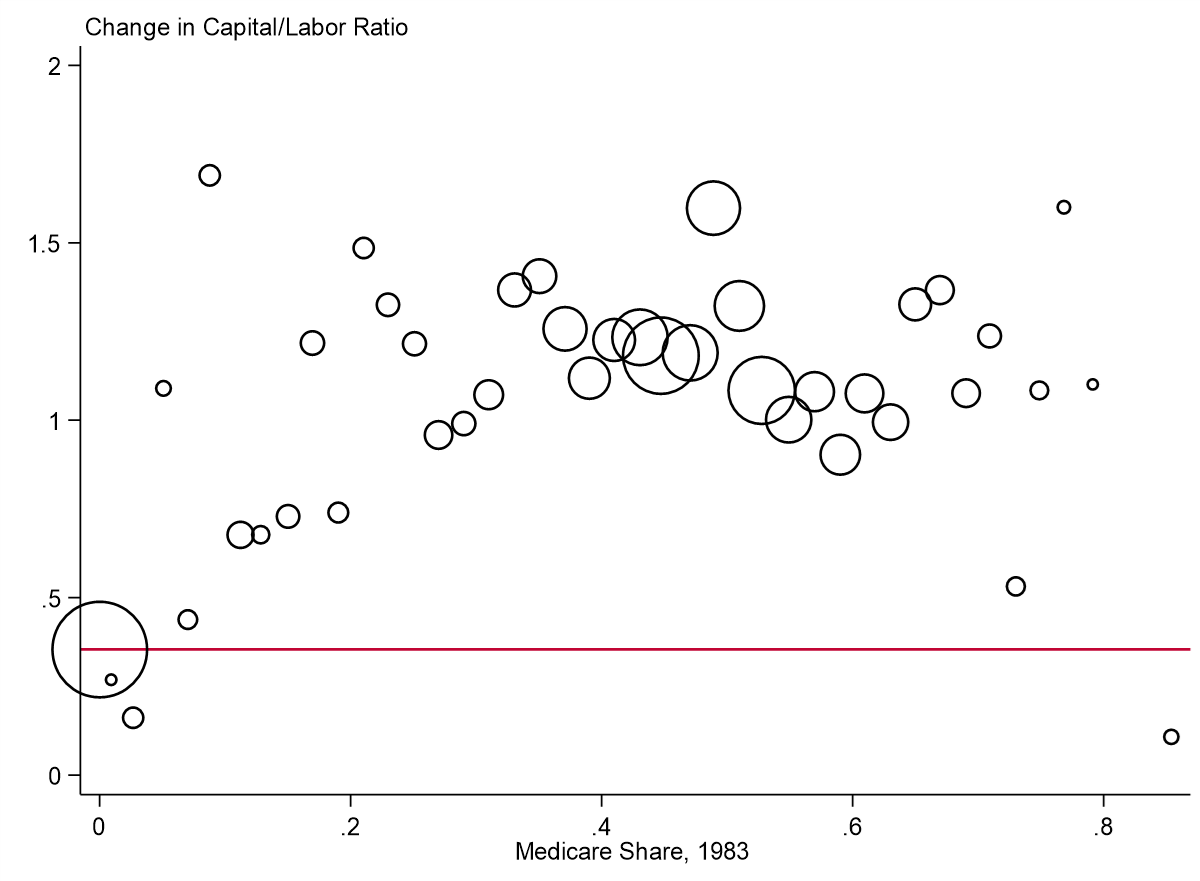## ATE Plot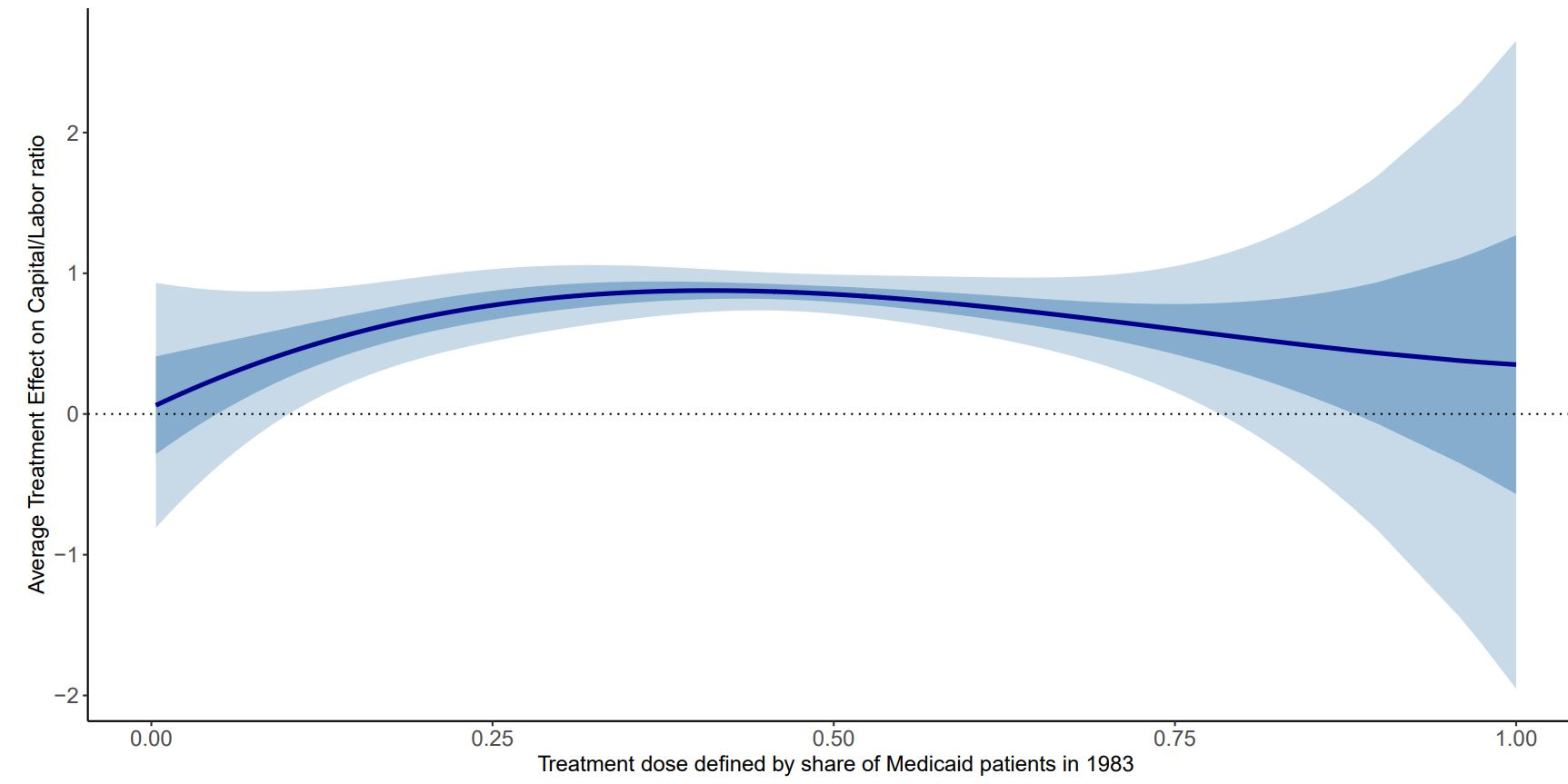## ACR(T) Plot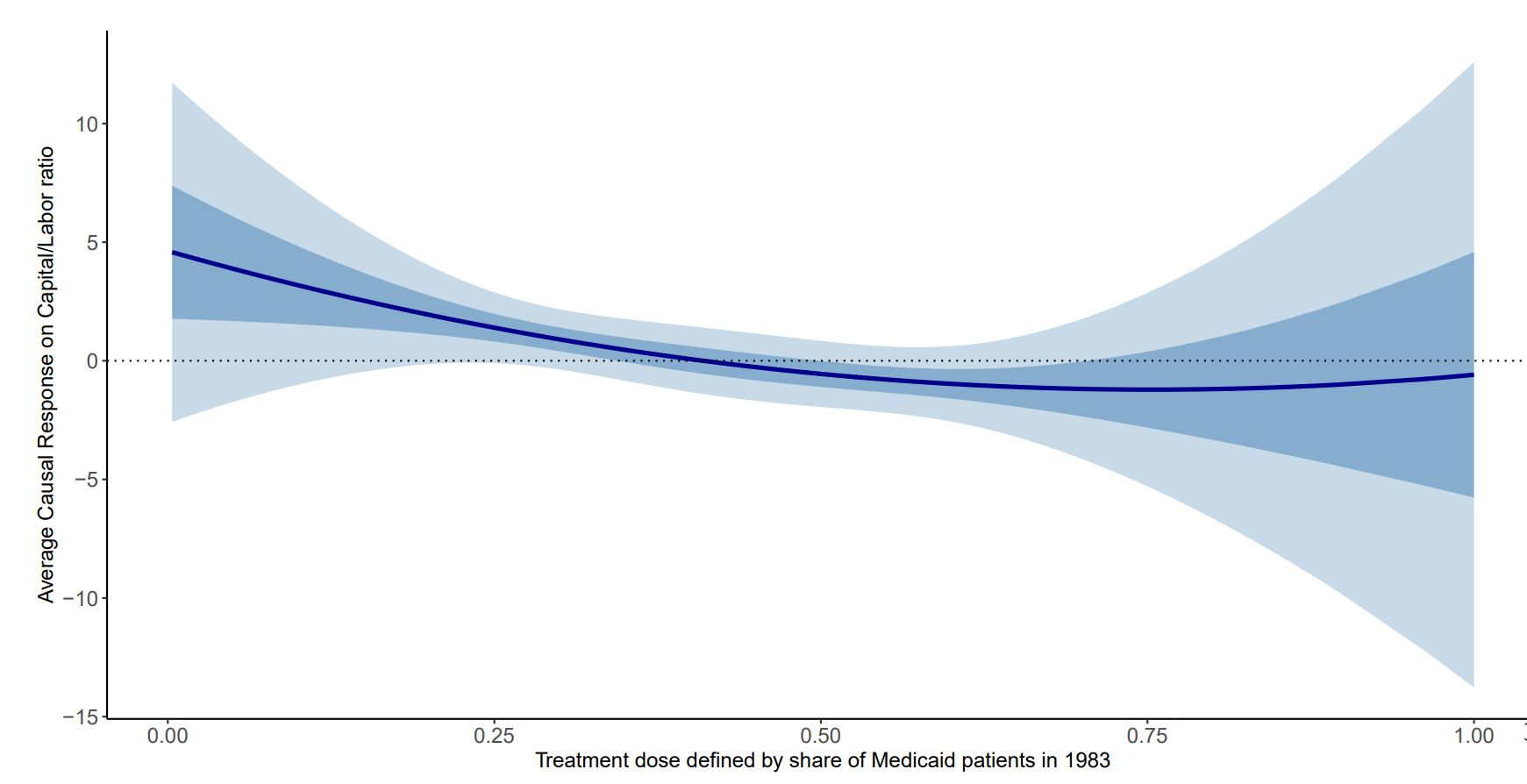## Results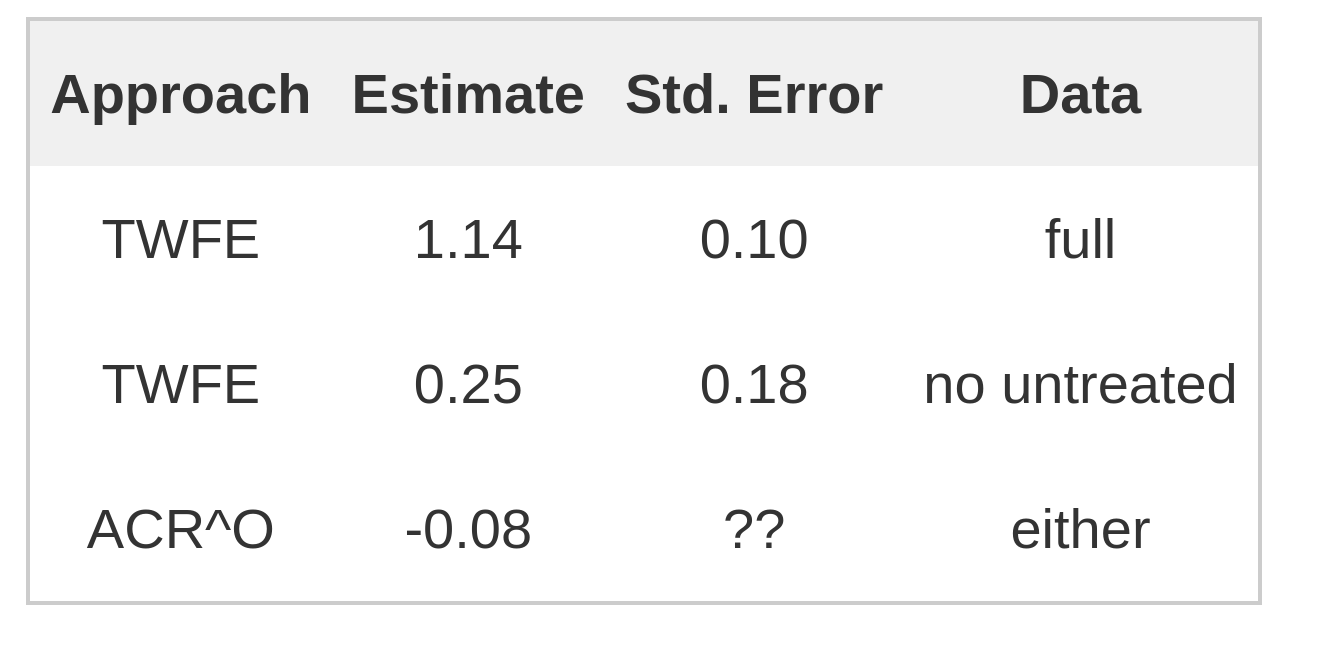## Density weights vs. TWFE weights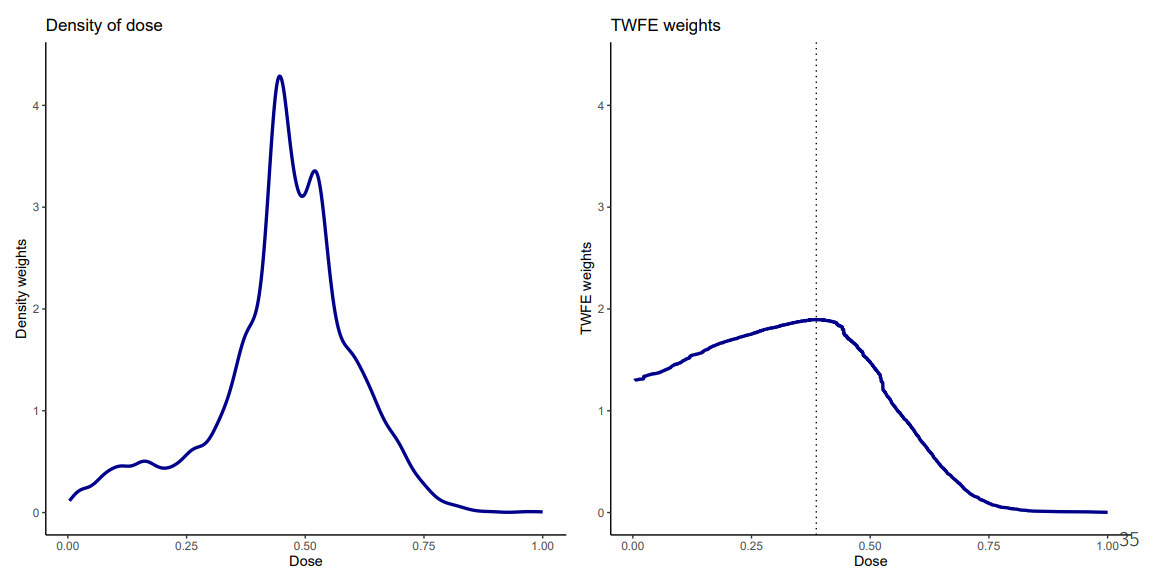## TWFE Weights with and without Untreated Group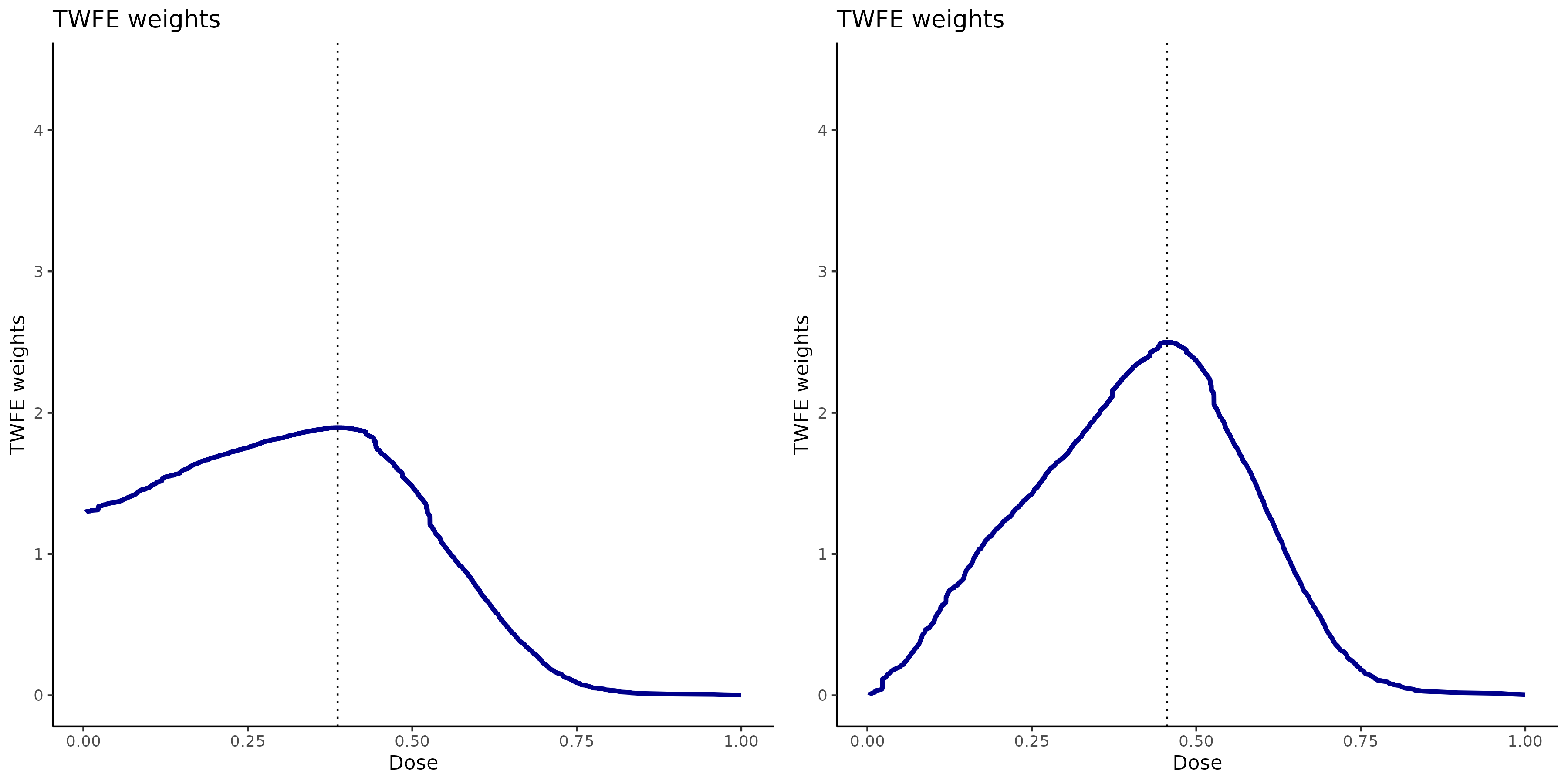Back

## Conclusion

• There are a number of challenges to implementing/interpreting DID with a continuous treatment

• The extension to multiple periods and variation in treatment timing is relatively straightforward (at least proceeds along the “expected” lines)

• But (in my view) the main new issue here is that justifying interpreting comparisons across different doses as causal effects requires stronger assumptions than most researchers probably think that they are making

• Other Summaries:   (i) Five minute summary       (ii) Pedro’s Twitter

• Code: in progress

Back

## Ex. Exponential Dose

Back

class: inverse, middle, center

## Setup

• If you are treated today, you will continue to be treated tomorrow

• Note relatively straightforward to relax, just makes notation more complex

• Can allow for treatment anticipation too, but ignoring for simplicity now

• Once become treated, dose remains constant (could probably relax this too)

## Setup

• $$G_i$$ — a unit’s “group” (the time period when unit becomes treated)

• Potential outcomes $$Y_{it}(g,d)$$ — the outcome unit $$i$$ would experience in time period $$t$$ if they became treated in period $$g$$ with dose $$d$$

• $$Y_{it}(0)$$ is the potential outcome corresponding to not being treated in any period

## Parameters of Interest

Level Effects:

$ATT(g,t,d|g,d) := \E[Y_t(g,d) - Y_t(0) | G=g, D=d] \ \ \ \textrm{and} \ \ \ ATE(g,t,d) := \E[Y_t(g,d) - Y_t(0) ]$ . . .

Slope Effects:

$ACRT(g,t,d|g,d) := \frac{\partial ATT(g,t,l|g,d)}{\partial l} \Big|_{l=d} \ \ \ \textrm{and} \ \ \ ACR(g,t,d) := \frac{\partial ATE(g,t,d)}{\partial d}$

## Parameters of Interest

These essentially inherit all the same issues as in the two period case

• Under a multi-period version of “standard” parallel trends, comparisons of $$ATT$$ across different values of dose are hard to interpret

• They contain selection bias terms
• Under a multi-period version of “strong” parallel trends, comparisons of $$ATE$$ across different values of dose straightforward to interpret

• But this involves a much stronger assumption

Expressions in remainder of talk are under “strong” parallel trends

• Under “standard” parallel trends, add selection bias terms everywhere

## Parameters of Interest

Often, these are high-dimensional and it may be desirable to “aggregate” them

• Average by group (across post-treatment time periods) and then across groups

$$\rightarrow$$ $$ACR^{overall}(d)$$ (overall average causal response for particular dose)

• Average $$ACR^{overall}(d)$$ across dose

$$\rightarrow$$ $$ACR^O$$ (this is just one number) and is likely to be the parameter that one would be targeting in a TWFE regression

• Event study: average across groups who have been exposed to treatment for $$e$$ periods

$$\rightarrow$$ For fixed $$d$$

$$\rightarrow$$ Average across different values of $$d$$ $$\implies$$ typical looking ES plot

class: inverse, middle, center

## TWFE Regression

Consider the same TWFE regression as before

$Y_{it} = \theta_t + \eta_i + \beta^{twfe} \cdot D_i \cdot Treat_{it} + v_{it}$